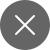# Understanding Cross Entropy Loss

4+
##### In this article, we will dive deep into cross-entropy and understand related topics too.

Don’t forget to check the confusion matrix and random forest also.

## What is Entropy?

We have heard this word many times. Simply put, it can be defined as some sort of randomness or disorder (according to thermodynamics). If we relate it from probability then, it can be stated as some sort of unpredictability or uncertainty.

In information theory, the entropy of a random variable or set of events is defined as the average level of information or uncertainty deep-rooted in the variable’s possible outcomes. In more simple terms, the more deterministic as even is, the less informative it will be.  Claude E. Shannon, the father of Information theory, had derived the relationship between the probability of n events and the entropy in the mathematical form by using the given equation.

Now, let’s see what Cross-Entropy is.

### Cross-Entropy

It is commonly used in machine learning as a loss or cost function. It is built upon entropy and calculates the difference between probability distributions. It can be considered as calculating total entropy between the probability distribution. It helps us to understand how we can minimize the loss to get better model performance.

Let us consider the occurrence of a particular event is represented by p. However, some machine learning models are predicted as q.

So, the cross-entropy for two probability distributions can be represented as –

Note: The value of entropy is always less than cross-entropy. Cross entropy will be equal to entropy when actual and predicted distribution is the same i.e., p=q

Here, the red wave is denoting the actual probability distribution whereas the orange line wave shows the predicted probability. The blue function is showing the cross-entropy between the two distributions. For proper visualization, refer to this.

It can be easily noticed from the graph that as the actual probability is moving away from the predicted one, there’s an increase in cross-entropy. So, we have to keep this distribution deviation as minimum as possible to reduce the cross-entropy.

### Cross-Entropy as a Loss function

Cross-Entropy works as a loss or cost function for the model predicting the probability value. It is mostly preferred in logistic regression and neural networks. Simply put, it is an optimization error function for training classification models that classifies the data by estimating the probability.

If the predicted probability of a class is different from the actual probability distribution, the value of cross-entropy will be high. If they both(predicted and actual probability)  are close to each other, cross-entropy will below. Cross entropy loss is more preferred than a mean squared error as it takes less training time and results in improved generalization. We can use a gradient descent algorithm along with a cross-entropy loss function to estimate model parameters.

Cross entropy loss for actual probability ‘y’ and estimated probability ‘p’ can be represented as the following  –

It can also be said as Log-loss. For calculating p, we can prefer the sigmoid function.

To make things more clear let’s visually see the relationship between estimated probability and cross-entropy.

It can be easily observed from plot 1, if the estimated probability of a true class is getting close to zero, the loss is increasing exponentially.

Plot 2 makes things more clear for us.

• When the actual label(red) is 1 and the predicted probability is also 1, the cost function near zero. Also, when the predicted value or hypothesis value is 0 cost is very high (close to infinite).
• When the actual label (green) is 0 and the predicted probability is 1, the cost function is very high(close to infinite). However, if the predicted value is 0, the cost is very less i.e., close to zero.

### Python Implementation

Let’s see how we can find the error for a classification problem using python.

Result:

Let’s discuss the different types of cross-entropy loss functions provided by Keras.

Binary cross-entropy:

It is used as a loss function for binary classification problems.
This cost function evaluates the loss between actual and predicted values.

Categorical cross-entropy:

It is used as a loss function for multi-class classification problems i.e. when we are having two or more target classes. As we are dealing with multiple classes we can use a one-hot encoding.

Sparse categorical cross-entropy: This loss function is somewhat similar to categorical cross-entropy. Here, target classes are represented  as using integer value – 0,1,2,3 etc.4+

### 3 Responses

1.Raghu krishna says:

Nice explanation Saumya!

2+
1. December 26, 2020

[…] check about cross-validation and cross-entropy […]

0
2. January 18, 2021

[…] discussed assumptions of Logistic regression and cross-entropy loss in my previous […]

0